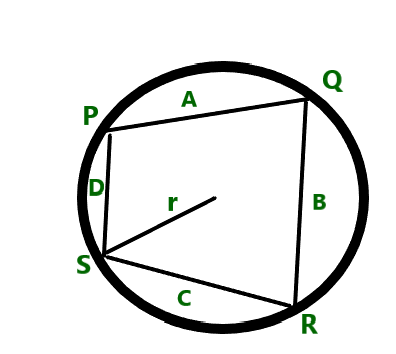GFG App
Open AppBrowser
Continue

# Calculate area of a cyclic quadrilateral with given side lengths

Given four positive integers A, B, C, and D representing the length of sides of a Cyclic Quadrilateral, the task is to find the area of the Cyclic Quadrilateral.

Examples:

Input: A = 10, B = 15, C = 20, D = 25
Output: 273.861

Input: A = 10, B = 30, C = 50, D = 20
Output: 443.706

Approach: The given problem can be solved based on the following observations:

• A cyclic quadrilateral is a quadrilateral whose vertices all lie on a single circle. The circle is called the circumcircle or circumscribed circle, and the vertices are said to be concyclic.• In the above image above r is the radius of the circumcircle and A, B, C, and D are the lengths of the sides PQ, QR, RS, and SP respectively.
• The area of the quadrilateral is given by Bretschneider’s formula is:where, A, B, C, and D are the sides of the triangle and
α and γ are the opposite angles of the quadrilateral.

Since, the sum of opposite angles of the quadrilateral is 180 degree. Therefore, the value of cos(180/2) = cos(90) = 0.

Therefore, the formula for finding the area reduces to.

Therefore, the idea is to print the value ofas the resultant area of the given quadrilateral.

Below is the implementation of the above approach:

## C++

 // C++ program for the above approach   #include  using namespace std;   // Function to find the area // of cyclic quadrilateral float calculateArea(float A, float B,                     float C, float D) {     // Stores the value of     // half of the perimeter     float S = (A + B + C + D) / 2;       // Stores area of cyclic quadrilateral     float area = sqrt((S - A) * (S - B)                       * (S - C) * (S - D));       // Return the resultant area     return area; }   // Driver Code int main() {     float A = 10;     float B = 15;     float C = 20;     float D = 25;     cout << calculateArea(A, B, C, D);       return 0; }

## Java

 // Java program for the above approach import java.io.*;   class GFG{       // Function to find the area // of cyclic quadrilateral static float calculateArea(float A, float B,                            float C, float D) {           // Stores the value of     // half of the perimeter     float S = (A + B + C + D) / 2;       // Stores area of cyclic quadrilateral     float area = (float)Math.sqrt((S - A) * (S - B) *                                    (S - C) * (S - D));       // Return the resultant area     return area; }   // Driver code public static void main (String[] args) {     float A = 10;     float B = 15;     float C = 20;     float D = 25;           System.out.println(calculateArea(A, B, C, D));   } }   // This code is contributed by Ankita saini

## Python3

 # Python3 program for the above approach from math import sqrt   # Function to find the area # of cyclic quadrilateral def calculateArea(A, B, C, D):           # Stores the value of     # half of the perimeter     S = (A + B + C + D) // 2       # Stores area of cyclic quadrilateral     area = sqrt((S - A) * (S - B) *                 (S - C) * (S - D))       # Return the resultant area     return area   # Driver Code if __name__ == '__main__':           A = 10     B = 15     C = 20     D = 25           print(round(calculateArea(A, B, C, D), 3))   # This code is contributed by mohit kumar 29

## C#

 // C# program for the above approach using System;   class GFG{   // Function to find the area // of cyclic quadrilateral static float calculateArea(float A, float B,                            float C, float D) {           // Stores the value of     // half of the perimeter     float S = (A + B + C + D) / 2;       // Stores area of cyclic quadrilateral     float area = (float)Math.Sqrt((S - A) * (S - B) *                                    (S - C) * (S - D));       // Return the resultant area     return area; }   // Driver Code static public void Main() {     float A = 10;     float B = 15;     float C = 20;     float D = 25;           Console.Write(calculateArea(A, B, C, D)); } }   // This code is contributed by code_hunt

## Javascript

 

Output:

273.861

Time Complexity: O(1)
Auxiliary Space: O(1)

My Personal Notes arrow_drop_up